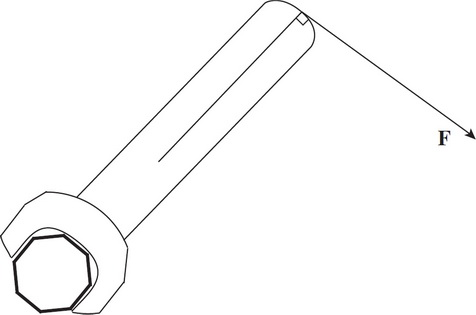# AP Physics 1 Question 234: Answer and Explanation

### Test Information

Question: 2344. In an effort to tighten a bolt, a force F is applied as shown in the figure above. If the distance from the end of the wrench to the center of the bolt is 20 cm and F = 20 N, what is the magnitude of the torque produced by F ?

• A. 1 N·m
• B. 2 N·m
• C. 4 N·m
• D. 10 N·m